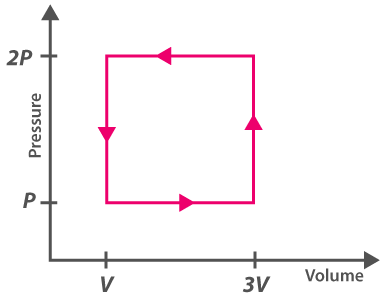# Physics Thermodynamics MCQ for NEET

NEET  Physics is the scoring paper in the medical entrance examination. Here, you will discover the NEET Physics MCQ Questions for all Concepts as per the latest syllabus. Practice more on a regular basis with these NEET Physics objective questions on air pollution and improve your subject knowledge & problem-solving skills along with time management. NEET Physics Physics Thermodynamics Multiple Choice Questions make you feel confident in answering the question in the exam & increases your scores to high.

## MCQ on Physics Thermodynamics

1. Following is the diagram of the thermodynamic process. Refer the diagram and match the followingP Process – I (a) Adiabatic
Q Process – II (b) Isobaric
R Process – III (c) Isochoric
S Process – IV (d) Isothermal
(a) (P,c), (Q,d), (R,b), (S,a)
(b) (P,c), (Q,d), (R,b), (S,a)
(c) (P,a), (Q,c), (R,d), (S,b)
(d) (P,c), (Q,a), (R,d), (S,b)

Answer: (d) (P,c), (Q,a), (R,d), (S,b)

2. Which of the following process is used to do maximum work done on the ideal gas if the gas is compressed to half of its initial volume?
(a) Isothermal
(b) Isochoric
(c) Isobaric

3. ABCDA is a cyclic process explaining the thermodynamic process. What is the work done by the system in the cycle(a) Zero
(b) $$\frac{\rho_{0} V_{0}}{2}$$
(c) ρ0V0
(d) 2ρ0V0

4. What is the ratio of $$\frac{C_{p}}{C_{v}}$$ for gas if the pressure of the gas is proportional to the cube of its temperature and the process is an adiabatic process.
(a) 2
(b) 3/2
(c) 4/3
(d) 5/3

5. The coefficient performance of a refrigerator is 5. If the temperature inside the freezer is -20℃, calculate the heat rejected to the surrounding
(a) 11℃
(b) 41℃
(c) 21℃
(d) 31℃

6. A thermodynamic system is explained with the help of the graph ABCD. What is the heat rejected by the system during the cycle?(a) pV
(b) 2pV
(c) 4pV
(d) 1/2pV

7. Select the incorrect statement
(a) For the triple point of water, 1°K is equal to 1/273.16.
(b) The first law of thermodynamics is also known as the law of thermal equilibrium
(c) The triple point of water is one of the reference points on the thermodynamic scale of temperature

8. What is a necessary condition for a reaction to be spontaneous at all temperatures?
(a) ΔH < ΔG
(b) ΔG and ΔH should be positive
(c) ΔH = ΔG = 0
(d) ΔG and ΔH should be negative

Answer: (d) ΔG and ΔH should be negative

9. A gas is compressed to half of its initial volume isothermally. The same gas is compressed again until the volume reduces to half through an adiabatic process. Then
(a) Work done during isothermal compression is more
(b) Work done is independent of the processes used for compression
(c) Work done is more during the adiabatic process
(d) Work done is dependent on the atomicity of the gas

10. Select the factor that affects the heat of reaction which is based on Kirchoff’s equation
(a) Molecularity
(b) Temperature
(c) Pressure
(d) Volume

11. For all the reactions what is the nature of the chemical dissociation?
(a) Exothermic
(b) Reversible
(c) Endothermic
(d) Reversible and endothermic